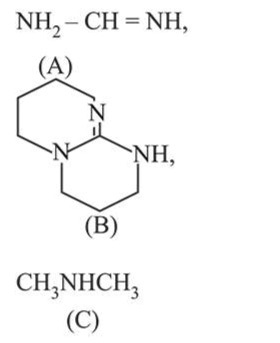# The increasing order ofQuestion:

The increasing order of $\mathrm{p} K_{\mathrm{b}}$ for the following compounds will be:1.  $(\mathrm{B})<(\mathrm{C})<(\mathrm{A})$

2. $(\mathrm{A})<(\mathrm{B})<(\mathrm{C})$

3.  $(\mathrm{C})<(\mathrm{A})<(\mathrm{B})$

4. $(\mathrm{B})<(\mathrm{A})<(\mathrm{C})$

Correct Option: , 4

Solution:

Conjugate acid of guanadine(B) is resonance stabilised and have 2 resonance structure.

Similarly conjugate acid of (A) is also resonance stabilised and have one resonance structure. (C) does not exhibit resonance structure.

therefore the basic order is, $k_{b}:(B)>(A)>(C)$

$\therefore \mathrm{pk}_{\mathrm{b}}:(\mathrm{B})<(\mathrm{A})<(\mathrm{C})$